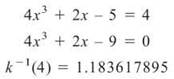﻿ ﻿THE DERIVATIVE OF AN INVERSE FUNCTION - Advanced Topics in Differentiation - AP CALCULUS AB & BC REVIEW - Master AP Calculus AB & BC

## Master AP Calculus AB & BC

Part II. AP CALCULUS AB & BC REVIEW

CHAPTER 5. Advanced Topics in Differentiation

OVERVIEW

• The derivative of an inverse function

• Hands-On Activity 5.1: Linear approximations

• L’Hopital’s Rule (BC topic only)

• Parametric derivatives (BC topic only)

• Polar derivatives (BC topic only)

• Technology: Finding polar and parametric derivatives with your calculator (BC topic only)

• Summing it up

The Power, Product, Quotient, and Chain Rules allow you to find the derivative of almost any expression or equation you will encounter. However, there are a few exceptions to the rule. This chapter serves to iron out those exceptions and make you invincible in the land of derivatives. In addition, BC students need to know how to differentiate polar, paramtetric, and vectorvalued equations, and these topics are included here.

THE DERIVATIVE OF AN INVERSE FUNCTION

Finding the inverse of a function is an important skill in mathematics (although you most certainly never have to use this skill in, say, the french fry department of the fast-food industry). Inverse functions are helpful for so many reasons; the main reason, of course, is their power to cause other functions to disappear. How do you solve the equation sec3x = 8? Find the cube root of each side to cancel the exponent and then take the arcsecant of each side to cancel the secant. (The answer, by the way, is π/3.) Functions this useful are bound to show up on the AP exam, and you should know how to differentiate them.

Example 1: If f(x) = (3x + 4)2, x ≥ -4/3 find (f-1)’(x).

Solution: Before you can find the derivative of the inverse, you need to find the inverse function. Use the method outlined in Chapter 2 as follows:Now, find (f-1)'(x):That was pretty easy, wasn’t it? However, as long as you’ve been in math classes, you’ve experienced teachers’ uncanny ability to take easy things and make them much more difficult—easy problems tend to leave you this uneasy feeling in the pit of your stomach like you’re in a slasher movie, and the killer is right behind you, but you’re afraid to turn around. In the case of inverse function differentiation, the killer takes the form of functions for which you cannot easily find an inverse.

How can you find the derivative of a function’s inverse if you can’t even find the inverse? You’ll need a formula based on a very simple characteristic of inverse functions: f(f-1(x)) = x. Take the derivative of the equation, using the Chain Rule, to getNow, solve the equation for (f-1)' (x):This is an incredibly useful formula to memorize; it’s especially handy when you cannot find an inverse function, as in the next example.

TIP. The formula for inverse derivatives can be remembered as 1/P.I., or “One over Prime/Inverse.” Many students remember that Magnum P.l. was a private inversetigator. Use the mnemonic device to remember the order.

Example 2: Find (g-1)' (3) if g(x) = x5 + 3x + 2.

Solution: If you try to find g-1(x) by switching the x and y and solving for y (as in Example 1), you get

x = y5 + 3y + 2

in which, despite your best efforts and lots of sweat, you cannot solve for y. Because you cannot easily find g-1(x), you should resort to the Magnum P.l. formula:or in this case:How are you going to find g-1(3) if you can’t find g-1(x)“? Good question. Time to be clever. If 3 is an input for g-1(x), then 3 must be an output of g(x), since the functions are inverses. Therefore, we should find the domain element x that results in an output of 3 for g(x):Solve this equation on your calculator, and the result is x = .33198902969. Thus, the point (.3319890296,3) falls on g(x), and resultingly, (3,.33 19890296) belongs on g-1(x). Did you miss it? You just found that g-1(3) = .3319890296. Return to the formula, and plug in what you know—the rest is easy:Because g'(x) = 5x4 + 3, g'(.3319890296) = 3.060738622 andThe process of finding derivatives of inverse functions becomes mechanical with practice. Even after the millionth problem like this, it still excites me to find values of an inverse function I don’t even know. What a rush!

EXERCISE 1

Directions: Solve each of the following problems. Decide which is the best of the choices given and indicate your responses in the book.

YOU MAY USE YOUR GRAPHING CALCULATOR FOR PROBLEM 2 ONLY.

1. If(a) Evaluate g-1(4) without finding g-1(x).

(b) Find g-1(x), and use it to verify your answer to part (a).

2. If k(x) = 4x3 + 2x - 5, find (k-1)'(4).

3. If h(x) is defined by the graph below, approximate (h-1)'(2).4. If m is a continuous and differentiable function with some values given by the table below, evaluate (m-1)’1/2.1. (a) To find g-1(4), set g(x) = 4 and solve for x.(b) Switch x and y and solve for y to get g-1(x):2. According to the formula,. Find k-1(4) using the same method as in 1(a):Because3. Again, the formula dictates thatFrom the graph, you can see that h-1(2) = 3 (the graph has an output of 2 when x = 3), so the formula becomesYou will have to approximate h'(3); the secant line connecting (2.5,1.5) to (3,2) looks like the best candidate. That secant line slope isso a good (and thankfully simple) approximation is h’(3) = 1. Therefore, you have4. The Magnum P.I. formula again rears its grotesque head:According to the table,(since m(—1) = —1/2). Also from the table, you see that m'(—1) = —2. Therefore,﻿